STAT 330: 96-3

Final Exam, 12 December 1996Instructor: Richard Lockhart

Instructions: This is an open book test. You may use notes, text, other books and a calculator. Your presentations of statistical analysis will be marked for clarity of explanation. I expect you to explain what assumptions you are making and to comment if those assumptions seem unreasonable. The exam is out of 75.

1. The Intelligence Quotient, or IQ, as measured by a certain psychological test is supposed to have a population distribution with mean 100 and standard deviation 15. Suppose I suspected that the mean IQ of children in Vancouver city public schools is higher than 100. How large a sample of children should I draw to give me at least a 90% chance of concluding that my suspicion is correct if the true mean IQ of such Vancouver children is 105. Assume that any test will be conducted at the level 5%. Make whatever assumptions are necessary but be sure to explain these assumptions. [5 marks]

Solution: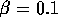,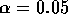, one sided,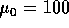,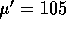and use the formula on page 319.

2. Two materials, called A and B for making the soles of shoes are to be compared. For each of 10 boys a coin is tossed to determine which sole (left or right shoe) to make out of material A, the other shoe getting material B. Outputs A, B and C present different analyses of the data. The response variable is amount of wear, larger values corresponding to shoes which are more worn out.

1. Is there a difference in wear between the two sole materials? [5 marks]

Solution: Paired comparisons. Use C. Difference is not significant.

2. Give a 98% confidence interval for the difference in mean wear between material A and material B. [3 marks]

Solution: From output C: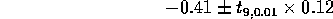3. Explain why each boy wore 1 shoe of each type rather than having 5 boys wear type A shoes on both feet and 5 boys wear type B. [1 mark]

Solutions: The paired comparisons design is more efficient if there is a strong correlation between the wear on the two feet of the same boy which would be expected.

4. What would be wrong with putting A on left shoes and B on the right on each boy? [1 mark]

Solution: You wouldn't be certain that a difference was really due to the different soles rather than due to the fact that the dominant foot (usually right) has a different wear pattern than the other foot.

3. The attached SAS output, D, shows parts of the input and output for the analysis of the results of the following experiment. A total of 48 plots of land were each fertilized with either fertilizer I, II or III - 16 plots of land receiving each fertilizer. Each plot was then planted with either seed type A or B or C or D. In each group of 16 plots 4 were chosen at random to plant with each seed type. Total yield was measured for each plot.

1. From the incomplete output produce a complete ANOVA table for the analysis of this data set. [5 marks]

Solution:

```                               Sum of      Mean   F
Source              DF        Squares    Square
FERT                2      5.22792917    2.614  46.93
SEED                3      3.56582292    1.188  21.33
Interaction         6      0.40427083    0.067   1.20
Error              36      1.95137500    0.0557
Corrected Total    47     11.14939792```
2. State and test relevant hypotheses, describing conclusions in real world terms in so far as possible. You will have to do fixed level testing in this problem. [5 marks]

Solution: The hypothesis of no interactions will be accepted while that of no main effect of SEED and that of no main effect of FERT are rejected at the 0.01 level.

3. Which fertilizer produces the highest yield? [2 marks]

Solution: Fertilizers I and II are indistinguishable from the Tukey intervals but both are better than III.

4. If I ran the analysis again without an interaction term what would the analysis of variance table be? You need not give the P-value column. [3 marks]

```                               Sum of      Mean   F
Source              DF        Squares    Square
FERT                2      5.22792917    2.614  46.60
SEED                3      3.56582292    1.188  21.18
Error              42      2.35564583    0.0561
Corrected Total    47     11.14939792```

4. One model for regression fits a straight line with no intercept. The model is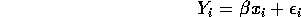where the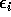are independent, have mean 0 and all have the same variance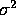which is unknown. There are n pairs with the numbers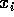for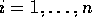being known values of some covariate. If this model is fitted by least squares, (that is by minimizing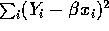) then the least squares estimate of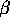is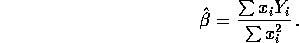However, an alternative estimate is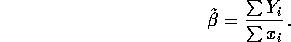1. Show that the estimator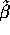is unbiased. [3 marks]

Solution: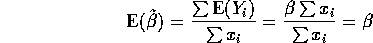2. Compute (give a formula for) the standard error of. [3 marks]

Solution: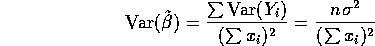3. Show that the mle ofin this model is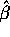, the least squares estimate, if thehave normal distributions. [4 marks]

Solution: The likelihood is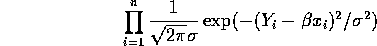and the log likelihood is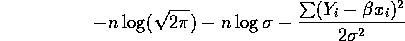The derivative with respect tois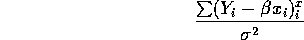which is 0 if and only if the numerator is 0 or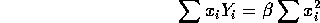Solving gives.

5. A certain machine is used to measure the breaking strength of samples of wire. However it is feared that measurements depend on the operator using the machine. Five batches of wire are made and each is split into 4 pieces. Each of the four pieces is randomly assigned to one of 4 machine operators, each operator getting one piece. The breaking strength of each piece is measured. Two possible analyses are SAS outputs E and F. Analyse the data in the most appropriate way, stating real world conclusions clearly and determining which operators are clearly different from which others. [10 marks]

Solution: This is a randomized complete blocks design for which the analysis is in output F. There is a clear difference between batches but no clear difference between operators.

6. Samples of 7 microwave ovens are obtained from each of 4 companies. The maximum power output in watts is measured for each oven. Use SAS output G.

1. Is there a difference between companies in mean power output? [5 marks]

Solution: The P value is 0.0001 so there is very clearly a difference between companies.

2. Is the mean power output for company B under 550 watts? [5 marks]

Solution: This is a one sample hypothesis testing problem. The output should have given means and SDs for the responses for the three companies so you could do this quickly and easily.

3. Give a 90% confidence interval for the ratio of population standard deviations for companies C and D. [5 marks]

Solution: The interval is based on the ratio of sample standard deviations and uses the F distribution.

7. The yield of a chemical reaction is measured 3 times at each of four temperatures. A simple linear regression is run; see output H.

1. Give a 95% confidence interval for the slope. [5 marks]

Solution: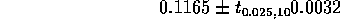2. Give a 90% confidence interval for the average yield at 200 degrees. [5 marks]

Solution: The predicted yield at 200 degrees is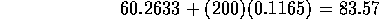The formula for the estimated standard error of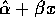is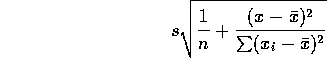The output gives s=0.62177 and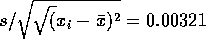. You need to compute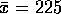and then you can use these numbers to figure out the standard error and get a confidence interval.

DATA

```  A    B   A shoe
13.2  14.0  L
8.2   8.8  L
10.9  11.2  R
14.3  14.2  L
10.7  11.8  R
6.6   6.4  L
9.5   9.8  L
10.8  11.3  L
8.8   9.3  R
13.3  13.6  L```
CODE
```  options pagesize=60 linesize=80;
data shoes;
infile 'shoes.dat';
input A B Ashoe \$ ;
proc glm  data=shoes;
model B = A;
run;```
OUTPUT
```                        General Linear Models Procedure
Dependent Variable: B
Sum of            Mean
Source                  DF          Squares          Square   F Value     Pr > F
Model                    1      55.74764638     55.74764638    333.73     0.0001
Error                    8       1.33635362      0.16704420
Corrected Total          9      57.08400000

R-Square             C.V.        Root MSE               B Mean
0.976590         3.702087       0.4087104            11.040000
Source                  DF      Type III SS     Mean Square   F Value     Pr > F
A                        1      55.74764638     55.74764638    333.73     0.0001

T for H0:    Pr > |T|   Std Error of
Parameter                  Estimate    Parameter=0                Estimate

INTERCEPT               0.247447347           0.41     0.6931     0.60475347
A                       1.015291877          18.27     0.0001     0.05557678```

DATA

```13.2  A
14.0  B
8.2  A
8.8  B
10.9  A
11.2  B
14.3  A
14.2  B
10.7  A
11.8  B
6.6  A
6.4  B
9.5  A
9.8  B
10.8  A
11.3  B
8.8  A
9.3  B
13.3  A
13.6  B```
CODE
```  data shoes;
infile 'shoes1.dat';
input wear material ;
proc sort data=shoes;
by material;
proc ttest cochran;
class material;
run;```
OUTPUT
```                                TTEST PROCEDURE
Variable: WEAR
MATERIAL       N                 Mean              Std Dev            Std Error
A             10          10.63000000           2.45132617           0.77517740
B             10          11.04000000           2.51846514           0.79640861

Variances        T    Method              DF    Prob>|T|
Unequal    -0.3689    Satterthwaite     18.0      0.7165
Cochran            9.0      0.7207
Equal      -0.3689                      18.0      0.7165

For H0: Variances are equal, F' = 1.06    DF = (9,9)    Prob>F' = 0.9372```

DATA

`As in A`
CODE
```  data shoes;
infile 'shoes.dat';
input A B Ashoe \$;
diff=A-B;
proc means mean std stderr t prt maxdec=2;
run;```
OUTPUT
```                                TTEST PROCEDURE
Variable: WEAR

MATERIAL       N                 Mean              Std Dev            Std Error
A             10          10.63000000           2.45132617           0.77517740
B             10          11.04000000           2.51846514           0.79640861

Variances        T    Method              DF    Prob>|T|
Unequal    -0.3689    Satterthwaite     18.0      0.7165
Cochran            9.0      0.7207
Equal      -0.3689                      18.0      0.7165

For H0: Variances are equal, F' = 1.06    DF = (9,9)    Prob>F' = 0.9372
Variable          Mean       Std Dev     Std Error             T  Prob>|T|
A                10.63          2.45          0.78         13.71    0.0001
B                11.04          2.52          0.80         13.86    0.0001
DIFF             -0.41          0.39          0.12         -3.35    0.0085```

DATA

```  8.83   I  A
9.20   I  A
9.22   I  A
9.16   I  A
9.80   I  B
10.10   I  B
9.87   I  B
9.67   I  B
9.16   I  C
9.20   I  C
9.54   I  C
9.73   I  C
9.20   I  D
9.66   I  D
9.58   I  D
9.52   I  D
8.98  II  A
8.76  II  A
9.08  II  A
8.53  II  A
9.92  II  B
9.51  II  B
9.29  II  B
10.22  II  B
9.18  II  C
8.95  II  C
8.83  II  C
9.08  II  C
9.42  II  D
10.02  II  D
9.66  II  D
9.03  II  D
8.49 III  A
8.44 III  A
8.29 III  A
8.53 III  A
8.80 III  B
9.01 III  B
9.03 III  B
8.76 III  B
8.53 III  C
8.61 III  C
8.57 III  C
8.49 III  C
8.80 III  D
8.98 III  D
8.83 III  D
8.89 III  D```
CODE
```  data yield;
infile 'seedfert.dat';
input yield fert \$ seed \$ ;
proc glm data=yield;
class fert seed;
model yield = fert|seed;
means  fert / tukey cldiff alpha=0.05;
means seed / tukey ;
run;```
OUTPUT
```                        General Linear Models Procedure
Dependent Variable: YIELD
Sum of            Mean
Source                  DF          Squares          Square
FERT                             5.22792917
SEED                             3.56582292
0.40427083
Error                            1.95137500
Corrected Total                 11.14939792
Tukey's Studentized Range (HSD) Test for variable: YIELD
Alpha= 0.05  Confidence= 0.95  df= 36  MSE= 0.054205
Critical Value of Studentized Range= 3.457
Minimum Significant Difference= 0.2012

Comparisons significant at the 0.05 level are indicated by '***'.

Simultaneous            Simultaneous
Lower    Difference     Upper
FERT        Confidence    Between   Confidence
Comparison        Limit       Means       Limit

I    - II       -0.01495     0.18625     0.38745
I    - III       0.57317     0.77438     0.97558   ***

II   - I        -0.38745    -0.18625     0.01495
II   - III       0.38692     0.58812     0.78933   ***

III  - I        -0.97558    -0.77438    -0.57317   ***
III  - II       -0.78933    -0.58812    -0.38692   ***

Tukey's Studentized Range (HSD) Test for variable: YIELD
Alpha= 0.05  df= 36  MSE= 0.054205
Critical Value of Studentized Range= 3.809
Minimum Significant Difference= 0.256
Means with the same letter are not significantly different.

Tukey Grouping              Mean      N  SEED

A           9.49833     12  B
A
A           9.29917     12  D

B           8.98917     12  C
B
B           8.79250     12  A```

DATA

```89	1	A
88	1	B
97	1	C
94	1	D
84	2	A
77	2	B
92	2	C
79	2	D
81	3	A
87	3	B
87	3	C
85	3	D
87	4	A
92	4	B
89	4	C
84	4	D
79	5	A
81	5	B
80	5	C
88	5	D```
CODE
```  data strength;
infile 'bhhp281q1.dat';
input strength batch \$ operator \$ ;
proc glm data=strength;
class operator;
model yield = operator;
means operator / tukey cldiff alpha=0.05;
run;```
OUTPUT
```                        General Linear Models Procedure
Dependent Variable: STRENGTH
Sum of            Mean
Source                  DF          Squares          Square   F Value     Pr > F
Model                    3      70.00000000     23.33333333      0.76     0.5318
Error                   16     490.00000000     30.62500000
Corrected Total         19     560.00000000
R-Square             C.V.        Root MSE        STRENGTH Mean
0.125000         6.434867       5.5339859            86.000000
Source                  DF      Type III SS     Mean Square   F Value     Pr > F
OPERATOR                 3      70.00000000     23.33333333      0.76     0.5318

Tukey's Studentized Range (HSD) Test for variable: STRENGTH
Alpha= 0.05  Confidence= 0.95  df= 16  MSE= 30.625
Critical Value of Studentized Range= 4.046
Minimum Significant Difference= 10.014

Comparisons significant at the 0.05 level are indicated by '***'.

Simultaneous            Simultaneous
Lower    Difference     Upper
OPERATOR      Confidence    Between   Confidence
Comparison        Limit       Means       Limit

C    - D          -7.014       3.000      13.014
C    - B          -6.014       4.000      14.014
C    - A          -5.014       5.000      15.014

D    - C         -13.014      -3.000       7.014
D    - B          -9.014       1.000      11.014
D    - A          -8.014       2.000      12.014

B    - C         -14.014      -4.000       6.014
B    - D         -11.014      -1.000       9.014
B    - A          -9.014       1.000      11.014

A    - C         -15.014      -5.000       5.014
A    - D         -12.014      -2.000       8.014
A    - B         -11.014      -1.000       9.014```

CODE

```  data strength;
infile 'bhhp281q1.dat';
input strength batch \$ operator \$ ;
proc glm data=strength;
class batch operator;
model yield = batch operator;
means batch / tukey cldiff alpha=0.05;
means operator / tukey cldiff alpha=0.05;
run;```
OUTPUT
```                        General Linear Models Procedure
Dependent Variable: STRENGTH
Sum of            Mean
Source                  DF          Squares          Square   F Value     Pr > F
Model                    7     334.00000000     47.71428571      2.53     0.0754
Error                   12     226.00000000     18.83333333
Corrected Total         19     560.00000000
R-Square             C.V.        Root MSE        STRENGTH Mean
0.596429         5.046208       4.3397389            86.000000
Source                  DF      Type III SS     Mean Square   F Value     Pr > F
BATCH                    4     264.00000000     66.00000000      3.50     0.0407
OPERATOR                 3      70.00000000     23.33333333      1.24     0.3387

Tukey's Studentized Range (HSD) Test for variable: STRENGTH
Alpha= 0.05  Confidence= 0.95  df= 12  MSE= 18.83333
Critical Value of Studentized Range= 4.508
Minimum Significant Difference= 9.781

Comparisons significant at the 0.05 level are indicated by '***'.

Simultaneous            Simultaneous
Lower    Difference     Upper
BATCH        Confidence    Between   Confidence
Comparison        Limit       Means       Limit

1    - 4          -5.781       4.000      13.781
1    - 3          -2.781       7.000      16.781
1    - 2          -0.781       9.000      18.781
1    - 5           0.219      10.000      19.781   ***

4    - 1         -13.781      -4.000       5.781
4    - 3          -6.781       3.000      12.781
4    - 2          -4.781       5.000      14.781
4    - 5          -3.781       6.000      15.781

3    - 1         -16.781      -7.000       2.781
3    - 4         -12.781      -3.000       6.781
3    - 2          -7.781       2.000      11.781
3    - 5          -6.781       3.000      12.781

2    - 1         -18.781      -9.000       0.781
2    - 4         -14.781      -5.000       4.781
2    - 3         -11.781      -2.000       7.781
2    - 5          -8.781       1.000      10.781

5    - 1         -19.781     -10.000      -0.219   ***
5    - 4         -15.781      -6.000       3.781
5    - 3         -12.781      -3.000       6.781
5    - 2         -10.781      -1.000       8.781

Tukey's Studentized Range (HSD) Test for variable: STRENGTH
Alpha= 0.05  Confidence= 0.95  df= 12  MSE= 18.83333
Critical Value of Studentized Range= 4.199
Minimum Significant Difference= 8.1485

Comparisons significant at the 0.05 level are indicated by '***'.

Simultaneous            Simultaneous
Lower    Difference     Upper
OPERATOR      Confidence    Between   Confidence
Comparison        Limit       Means       Limit

C    - D          -5.149       3.000      11.149
C    - B          -4.149       4.000      12.149
C    - A          -3.149       5.000      13.149

D    - C         -11.149      -3.000       5.149
D    - B          -7.149       1.000       9.149
D    - A          -6.149       2.000      10.149

B    - C         -12.149      -4.000       4.149
B    - D          -9.149      -1.000       7.149
B    - A          -7.149       1.000       9.149

A    - C         -13.149      -5.000       3.149
A    - D         -10.149      -2.000       6.149
A    - B          -9.149      -1.000       7.149```

DATA

```560 1
546 1
547 1
548 1
559 1
559 1
544 1
477 2
468 2
523 2
484 2
524 2
527 2
457 2
455 3
481 3
506 3
492 3
468 3
450 3
448 3
460 4
503 4
482 4
526 4
462 4
545 4
534 4```
```  data wattage;
infile 'erglep656q17.dat';
input watts  company  ;
proc sort data=wattage;
by company;
proc glm  data=wattage;
class company;
model watts = company;
means company / tukey cldiff;
run;```
OUTPUT
```                        General Linear Models Procedure
Dependent Variable: WATTS
Sum of            Mean
Source                  DF          Squares          Square   F Value     Pr > F
Model                    3     24136.678571     8045.559524     12.24     0.0001
Error                   24     15779.428571      657.476190
Corrected Total         27     39916.107143
R-Square             C.V.        Root MSE           WATTS Mean
0.604685         5.079281       25.641299            504.82143

Tukey's Studentized Range (HSD) Test for variable: WATTS
Alpha= 0.05  Confidence= 0.95  df= 24  MSE= 657.4762
Critical Value of Studentized Range= 3.901
Minimum Significant Difference= 37.809

Comparisons significant at the 0.05 level are indicated by '***'.

Simultaneous            Simultaneous
Lower    Difference     Upper
COMPANY       Confidence    Between   Confidence
Comparison        Limit       Means       Limit

1    - 4           12.33       50.14       87.95   ***
1    - 2           19.76       57.57       95.38   ***
1    - 3           42.62       80.43      118.24   ***

4    - 1          -87.95      -50.14      -12.33   ***
4    - 2          -30.38        7.43       45.24
4    - 3           -7.52       30.29       68.09

2    - 1          -95.38      -57.57      -19.76   ***
2    - 4          -45.24       -7.43       30.38
2    - 3          -14.95       22.86       60.67

3    - 1         -118.24      -80.43      -42.62   ***
3    - 4          -68.09      -30.29        7.52
3    - 2          -60.67      -22.86       14.95```

DATA

```150 77.4
150 76.7
150 78.2
200 84.1
200 84.5
200 83.7
250 88.9
250 89.2
250 89.7
300 94.8
300 94.7
300 95.9```
CODE
```  data yield;
infile 'yield.dat';
input temp yield ;
proc glm  data=yield;
model yield = temp;
run;```
OUTPUT
```                        General Linear Models Procedure
Dependent Variable: YIELD
Sum of            Mean
Source                  DF          Squares          Square   F Value     Pr > F
Model                    1     509.25066667    509.25066667   1317.25     0.0001
Error                   10       3.86600000      0.38660000
Corrected Total         11     513.11666667
R-Square             C.V.        Root MSE           YIELD Mean
0.992466         0.718950       0.6217717            86.483333
Source                  DF      Type III SS     Mean Square   F Value     Pr > F
TEMP                     1     509.25066667    509.25066667   1317.25     0.0001

T for H0:    Pr > |T|   Std Error of
Parameter                  Estimate    Parameter=0                Estimate
INTERCEPT               60.26333333          80.96     0.0001     0.74439685
TEMP                     0.11653333          36.29     0.0001     0.00321082```